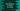# Write a C program to find the frequency of vowels in a stringLet’s write a C program to find the frequency of vowels in a string. This program will ask the user to enter a string, read it and print out the count of each vowel. The calculation will be case-insensitive i.e. the vowel can be either upper case or lower case.

## Algorithm :

We will use the below algorithm in this program :

1. Ask the user to enter a string.
2. Initialize five different variables to store the count of each vowel.
3. Iterate through the characters of that string one by one.
4. Check for each character, if it is a vowel or not. Also, check which vowel it is.
5. Increment the count for that corresponding vowel.

## C program :

``````#include <stdio.h>

int main()
{
char strArr;
int count_a = 0, count_e = 0, count_i = 0, count_o = 0, count_u = 0;

printf("Enter a string : ");
fgets(strArr, 100, stdin);

int i = 0;
while (strArr[i] != '\0')
{
switch (strArr[i])
{
case 'a':
count_a++;
break;
case 'A':
count_a++;
break;
case 'e':
count_e++;
break;
case 'E':
count_e++;
break;
case 'i':
count_i++;
break;
case 'I':
count_i++;
break;
case 'o':
count_o++;
break;
case 'O':
count_o++;
break;
case 'u':
count_u++;
break;
case 'U':
count_u++;
break;
}
i++;
}

printf("Count of A : %d\nCount of E : %d\nCount of I : %d\nCount of O : %d\nCount of U : %d\n", count_a, count_e, count_i, count_o, count_u);
return 0;
}``````

### Explanation :

Below are the explanation for the above program :

1. strArr is a character array of size 100. This character array is used to hold the user input string.
2. This program is taking the user input string using fgets and storing that value in strArr.
3. Using a while loop, we are iterating through the characters one by one.
4. Also, we are using one switch-case block to check the current iterating character. It checks if the character is a vowel or not. If it is a vowel, it increments the counter for that vowel.
5. If the current character is not vowel, it will not execute the switch-case block.
6. Finally, we are printing out the count of each vowel.

### Sample Output :

``````Enter a string : hello world
Count of A : 0
Count of E : 1
Count of I : 0
Count of O : 2
Count of U : 0

Enter a string : The quick brown fox jumps over the lazy dog
Count of A : 1
Count of E : 3
Count of I : 1
Count of O : 4
Count of U : 2``````